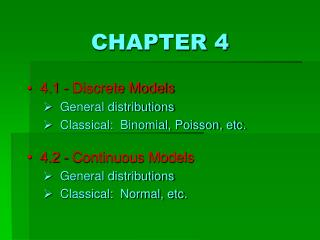DownloadDownload PresentationCHAPTER 4

# CHAPTER 4

Télécharger la présentation## CHAPTER 4

- - - - - - - - - - - - - - - - - - - - - - - - - - - E N D - - - - - - - - - - - - - - - - - - - - - - - - - - -
##### Presentation Transcript

1. CHAPTER 4 4.1 - Discrete Models General distributions Classical: Binomial, Poisson, etc. 4.2 - Continuous Models General distributions Classical: Normal, etc.

2. What is the connection between probability and random variables? Events (and their corresponding probabilities) that involve experimental measurements can be described by random variables(e.g., “X = # Males” in previous gender equity example).

3. POPULATION Discrete random variable X Example: X = Cholesterol level (mg/dL) x1 x3 x2 x6 x4 …etc…. x5 xn SAMPLE of size n

4. POPULATION Discrete random variable X Example: X = Cholesterol level (mg/dL) X Total Area = 1 Probability Histogram f(x)= “probability mass function” (pmf) Hey!!! What about the population mean  and the population variance  2 ??? Calculating probabilities… P(aX b) = ???????? f (x) | x | b | a

5. POPULATION Discrete random variable X Example: X = Cholesterol level (mg/dL) • Population mean Also denoted by E[X], the “expected value” of the variable X. • Population variance Just as the sample meanand sample variances2 were used to characterize “measure of center” and “measure of spread” of a dataset, we can now define the “true” population mean and population variance 2, using probabilities.

6. POPULATION Discrete random variable X Example: X = Cholesterol level (mg/dL) • Population mean Also denoted by E[X], the “expected value” of the variable X. • Population variance Just as the sample meanand sample variances2 were used to characterize “measure of center” and “measure of spread” of a dataset, we can now define the “true” population mean and population variance 2, using probabilities.

7. Example 1: POPULATION Discrete random variable X Example: X = Cholesterol level (mg/dL) 1/2 1/3 1/6 250 500

8. Example 2: POPULATION Discrete random variable X Example: X = Cholesterol level (mg/dL) Equally likelyoutcomes result in a “uniform distribution.” 1/3 1/3 1/3 (clear from symmetry) 210 600

9. To summarize…

10. Probability Table Probability Histogram POPULATION Total Area = 1 Discrete random variable X X Frequency Table Density Histogram x1 x3 x2 x6 x4 Total Area = 1 …etc…. x5 xn X SAMPLE of size n

11. Probability Table Probability Histogram POPULATION ? Total Area = 1 Discrete random variable X Continuous X Frequency Table Density Histogram x1 x3 x2 x6 x4 Total Area = 1 …etc…. x5 xn X SAMPLE of size n

12. Example 3: TWO INDEPENDENT POPULATIONS X1 = Cholesterol level (mg/dL) X2 = Cholesterol level (mg/dL) 2 = 210 22 = 600 1 = 250 12 = 500 NOTE: By definition, this is the sample space of the experiment! NOTE: By definition, this is the sample space of the experiment! What are the probabilities of the corresponding events “D = d” for d = -30, 0, 30, 60, 90? D = X1 – X2 ~ ???

13. Example 3: TWO INDEPENDENT POPULATIONS X1 = Cholesterol level (mg/dL) X2 = Cholesterol level (mg/dL) 2 = 210 22 = 600 1 = 250 12 = 500 The outcomes of Dare NOT EQUALLY LIKELY!!! D = X1 – X2 ~ ??? NO!!!

14. Example 3: TWO INDEPENDENT POPULATIONS X1 = Cholesterol level (mg/dL) X2 = Cholesterol level (mg/dL) 2 = 210 22 = 600 1 = 250 12 = 500 D = X1 – X2 ~ ???

15. Example 3: TWO INDEPENDENT POPULATIONS X1 = Cholesterol level (mg/dL) X2 = Cholesterol level (mg/dL) 2 = 210 22 = 600 1 = 250 12 = 500 D = X1 – X2 ~ ???

16. Example 3: TWO INDEPENDENT POPULATIONS Probability Histogram X1 = Cholesterol level (mg/dL) X2 = Cholesterol level (mg/dL) 2 = 210 22 = 600 1 = 250 12 = 500 6/18 5/18 3/18 3/18 1/18 D = X1 – X2 ~ ??? What happens if the two populations are dependent? SEE LECTURE NOTES!

17. Example 3: TWO INDEPENDENT POPULATIONS Probability Histogram X1 = Cholesterol level (mg/dL) X2 = Cholesterol level (mg/dL) 2 = 210 22 = 600 2 = 210 22 = 600 1 = 250 12 = 500 1 = 250 12 = 500 6/18 5/18 3/18 3/18 1/18 D = (-30)(1/18) + (0)(3/18) + (30)(6/18) + (60)(5/18) + (90)(3/18) = 40 D = X1 – X2 ~ ??? D = 1 – 2 D2 = (-70)2(1/18) + (-40)2(3/18) + (-10)2(6/18) + (20)2(5/18) + (50)2(3/18) = 1100 D2 = 12 + 22

18. General: TWO INDEPENDENT POPULATIONS IF the two populations are dependent… Probability Histogram X1 = Cholesterol level (mg/dL) X1 X2 = Cholesterol level (mg/dL) X2 …then this formula still holds, BUT…… 2 = 210 22 = 600 2 = 210 22 = 600 1 = 250 12 = 500 1 = 250 12 = 500 6/18 5/18 3/18 3/18 1/18 Mean (X1 – X2) = Mean (X1) – Mean (X2) D = (-30)(1/18) + (0)(3/18) + (30)(6/18) + (60)(5/18) + (90)(3/18) = 40 D = X1 – X2 ~ ??? D = 1 – 2 Var (X1 – X2) = Var (X1) + Var (X2) D2 = (-70)2(1/18) + (-40)2(3/18) + (-10)2(6/18) + (20)2(5/18) + (50)2(3/18) = 1100 – 2 Cov (X1, X2) These two formulas are valid for continuous as well as discrete distributions. D2 = 12 + 22